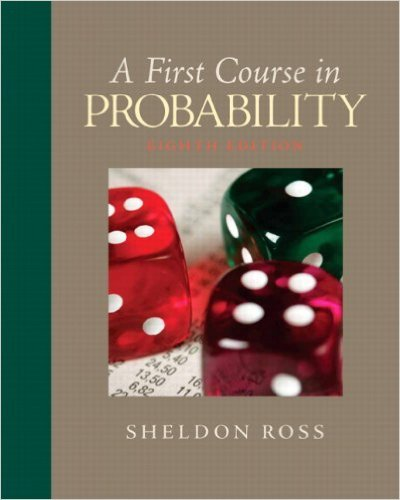×
×

# A box contains 3 marbles: 1 red, 1 green, and 1blue.ISBN: 9780136033134 163

## Solution for problem 1 Chapter 2

First Course in Probability | 8th Edition

• Textbook Solutions
• 2901 Step-by-step solutions solved by professors and subject experts
• Get 24/7 help from StudySoup virtual teaching assistantsFirst Course in Probability | 8th Edition

4 5 1 275 Reviews
20
2
Problem 1

A box contains 3 marbles: 1 red, 1 green, and 1blue. Consider an experiment that consists of taking1 marble from the box and then replacing itin the box and drawing a second marble from thebox. Describe the sample space. Repeat when thesecond marble is drawn without replacing the firstmarble.

Step-by-Step Solution:
Step 1 of 3

Review of Notes 4: i = 1, 2 ….. n j = 1, 2 ….r i ̅ ∑ ∑ ̅ ∑ ∑ ∑ ∑ ( ̅) => Overall Variation (SST total sum of squares) ∑ ∑ ( ) = {( ̅ ) ̅ ̅ } = ∑ ∑ ( ̅ ) ∑ ∑...

Step 2 of 3

Step 3 of 3

##### ISBN: 9780136033134

First Course in Probability was written by and is associated to the ISBN: 9780136033134. The full step-by-step solution to problem: 1 from chapter: 2 was answered by , our top Statistics solution expert on 11/23/17, 05:06AM. The answer to “A box contains 3 marbles: 1 red, 1 green, and 1blue. Consider an experiment that consists of taking1 marble from the box and then replacing itin the box and drawing a second marble from thebox. Describe the sample space. Repeat when thesecond marble is drawn without replacing the firstmarble.” is broken down into a number of easy to follow steps, and 49 words. Since the solution to 1 from 2 chapter was answered, more than 254 students have viewed the full step-by-step answer. This textbook survival guide was created for the textbook: First Course in Probability, edition: 8. This full solution covers the following key subjects: box, marble, replacing, Green, contains. This expansive textbook survival guide covers 10 chapters, and 495 solutions.

Unlock Textbook Solution